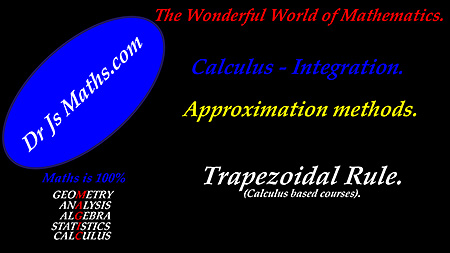Dr. J's Maths.com
Where the techniques of Maths
are explained in simple terms.

Calculus - Integration - Main Page.

As noted on the Calculus historical development page:

Leibniz began his development of Calculus from the study of areas and volumes.

Newton began his development of Calculus with the study of the continuous movement of a point
which led him to examine gradients of curves.

As they extended their work individually, both men saw the significant overlap in their theoretical work.

So integration was the field and the technique which was the first focus of development by Leibniz.

It is handy to remember these links:

Integration is used to calculate area and volume.

Differentiation is used to calculate gradients and slopes of curves.

Anti-differentiation.

The term anti-differentiation is synonymous with indefinite integration or primitive integration.
The technique of anti-differentiation is used when we want to find the function whose derivative is what we are now given.
Hence an anti-derivative is sometimes referred to as a primitive function or as an indefinite integral.

So if we have a function F(x), its derivative might be called f(x). So F '(x) = f(x).

Don't get too concerned about the distinction. Just think:
If we have F(x), its derivative is F '(x) which we can call f(x).
When we go backwards, then f(x) integrates to become the anti-derivative F(x).
If we substitute values for the original function F(x) into the anti-derivative, we can create the location constant.

From this page of the site, integration resources related to basic functions can be accessed. For trigonometric, exponential and logarithmic functions, access the separate main pages from the main menu.

 The resources which can be accessed directly are: 1. Approximation methods for finding areas or integrals. 3. Reverse Chain Rule questions.

 Learning area. Resource. 1. Approximation methods for finding areas and evaluating integrals. Area of a basic trapezium. See Trapezoidal Rule - Main Page. Trapezoidal Rule (non-calculus courses). See Trapezoidal Rule - Main Page. Trapezoidal Rule (Calculus courses).Review of finding the area of a trapezium. Review of the steps for applying the trapezoidal rule to find area or volume. Trapezoidal Rule - Test Yourself 1. Trapezoidal Rule - Test Yourself 1 - Solutions. 2. Basic strategies for integration (anti-differentiation).Steps to follow for basic integration. Basic integration - Test Yourself 1. Basic integration - Test Yourself 1 - Solutions. Basic integration - Test Yourself 2. Basic integration - Test yourself 2 - Solutions. 3. Integration by substitution - Reverse Chain Rule .Basic functions Concept of the technique and steps to follow when using the Reverse Chain Rule. Reverse Chain Rule - Test Yourself 1. Reverse Chain Rule - Test Yourself 1 - Solutions. Exponential functions Reverse Chain Rule - Exponentials - Test Yourself 1. Reverse Chain Rule - Exponentials - Test Yourself 1 - Solutions. Logarithmic functions Reverse Chain Rule - Logarithms - Test Yourself 1. Reverse Chain Rule - Logarithms - Test Yourself 1 - Solutions. Trigonometric functions. Reverse Chain Rule - Trig functions - Test Yourself 1. Reverse Chain Rule - Trig functions - Test Yourself 1 - Solutions. Multi-function. Reverse Chain Rule - Multi-function - Test Yourself 1. Reverse Chain Rule - Multi-function - Test Yourself 1 - Solutions. 4. Differentiate ... hence find.Concept of the technique and the steps to follow for these types of questions. Basic functions. Differentiate ... hence find - Test Yourself 1. Differentiate ... hence find - Test Yourself 1 - Solutions. Exponential functions. Differentiate (exp. function) ... hence find - Test Yourself 1. Differentiate (exp. function) ... hence find - Test Yourself 1 - Solutions. Logarithmic functions. Differentiate (log function) ... hence find - Test Yourself 1. Differentiate (log function) ... hence find - Test Yourself 1 - Solutions Trigonometric functions. Differentiate (trig function) ... hence find -Test Yourself 1. Differentiate (trig function) ... hence find - Test Yourself 1 - Solutions Mixed functions. Differentiate (mixed functions) ... hence find - Test Yourself 1. Differentiate (mixed functions) ... hence find - Test Yourself 1 - Solutions. 5. Other Integration by substitution - extension. Extended substitution - Test Yourself 1. Extended substitution - Test Yourself 1 - Solutions. 6. Applications including calculating areas with calculus.Steps to apply for finding areas under curves or between two curves. Integration - Areas - Test Yourself 1. Integration - Areas - Test Yourself 1 - solutions. Integration - Areas - Test Yourself 2. Integration - Areas - Test Yourself 2 - solutions.